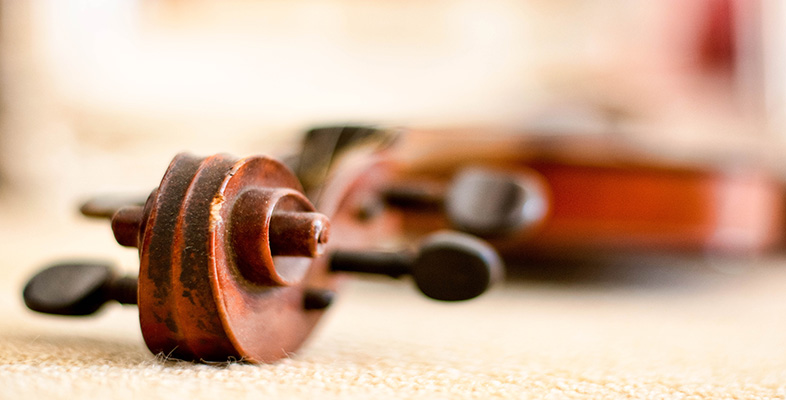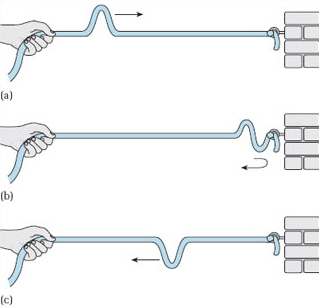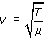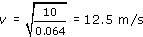Creating musical sounds

This free course is available to start right now. Review the full course description and key learning outcomes and create an account and enrol if you want a free statement of participation.

Free course

# 5.2 Vibrating string: speed of wave propagation

If standing waves are set up when two travelling waves moving in opposite directions interact, then how are standing waves set up on a string and why are they set up only at certain frequencies?

To help answer these questions, I want you first to imagine a length of string that is fixed at one end and held in someone's hand at the other. Suppose the person holding the string flicks their end of the string in such a way that an upward pulse is sent along the string.

As the pulse passes a given position, the string at that position is displaced first upwards and then down again (Figure 8(a)). The string displacement is perpendicular to the direction of propagation of the pulse. For this reason, waves on a string are said to be transverse.

When the pulse reaches the fixed end it is reflected (Figure 8(b)). The reflected pulse travels back towards the person holding the string and has exactly the same shape as the initial pulse except that it is upside down. It has been inverted and is now a downward pulse (Figure 8(c)).Figure 8 Reflection of a pulse on a string from a fixed end

## Activity 10

Run the Flash animation below. It shows a pulse travelling down a string, then being reflected and inverted at a fixed end.

Use the controls below (Play/Pause, Step, Reset) to run flash animation.

Active content not displayed. This content requires JavaScript to be enabled, and a recent version of Flash Player to be installed and enabled.
Interactive feature not available in single page view (see it in standard view).

The speed with which a pulse or a wave travels along the string is called the wave speed. It is measured in metres per second (m/s) and depends on both the tension of the string and its mass per unit length. Indeed, the wave speed v in a stretched string can be described by the following equation:where the tension T is measured in newtons (N) and the mass per unit length μ is measured in kilograms per metre (kg/m).

## Activity 11

Watch the video clip below. It shows a pulse travelling along a 5 m length of elastic rope, being reflected and inverted at the far end and returning back along the rope. The elasticity in the rope ensures that the pulse can be seen clearly. Note that the time the pulse takes to travel to the end of the rope and back again is 0.8 second.

Download this video clip.Video player: ta212_2_001v.mp4
Interactive feature not available in single page view (see it in standard view).

## Activity 12

• (a) Using the time you noted in Activity 11, and remembering that the rope is 5 m long, calculate the speed at which the pulse travels along the rope.

• (b) When the video in Activity 11 was filmed, the rope was being stretched so that its tension was 10N. The total mass of the rope was 0.32 kg. Using the wave speed equation given above, calculate the speed at which the pulse travels along the rope. Compare your answer with the speed that you calculated in part (a).

### Answer

• (a) The pulse takes 0.8 second to travel down the rope and back. That is, it takes 0.8 second to travel 10 m. Therefore it travels at a speed of v = 10/0.8 = 12.5 m/s.

• (b)The mass per unit length μ of the rope is simply the mass that a 1 m length of the rope would have. So, if a 5 m length has a mass of 0.32 kg, a 1 m length will have a mass of 0.32/5 = 0.064 kg. Therefore the mass per unit length μ = 0.064 kg/m. The tension T = 10 N, so substituting the values for T and μ into the wave speed equation gives:The wave speed predicted by the wave speed equation matches that calculated in part (a).

TA212_2

### Take your learning further

Making the decision to study can be a big step, which is why you'll want a trusted University. The Open University has 50 years’ experience delivering flexible learning and 170,000 students are studying with us right now. Take a look at all Open University courses.

If you are new to university level study, find out more about the types of qualifications we offer, including our entry level Access courses and Certificates.

Not ready for University study then browse over 900 free courses on OpenLearn and sign up to our newsletter to hear about new free courses as they are released.

Every year, thousands of students decide to study with The Open University. With over 120 qualifications, we’ve got the right course for you.

Request an Open University prospectus